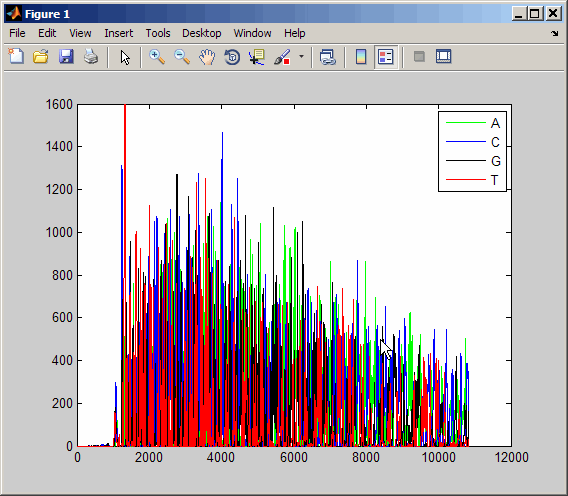# traceplot

Draw nucleotide trace plots

## Syntax

```traceplot(TraceStructure) traceplot(A, C, G, T) h = traceplot(...) ```

## Description

`traceplot(TraceStructure)` creates a trace plot from data in a structure with fields `A`, `C`, `G`, and `T`.

`traceplot(A, C, G, T)` creates a trace plot from data in vectors `A`, `C`, `G`, and `T`.

`h = traceplot(...)` returns a structure with the handles of the lines corresponding to `A`, `C`, `G`, `T`.

## Examples

1. Read trace data from an SCF-formatted file into a MATLAB® structure.

```tstruct = scfread('sample.scf') tstruct = A: [10827x1 double] C: [10827x1 double] G: [10827x1 double] T: [10827x1 double]```
2. Draw a nucleotide trace plot of the data.

`traceplot(tstruct)`## Version History

Introduced before R2006a